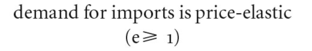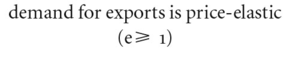# Marshall-Lerner condition

(redirected from Marshall-Lerner)

## Marshall-Lerner Condition

In international trade, a theory stating that if the sum of price elasticity of a country's exports and the price elasticity of its imports is greater than one, a devaluation of that country's currency will improve its balance of trade. Devaluation does not improve the balance of trade if the sum is any lower.

## Marshall-Lerner condition

the PRICE ELASTICITY OF DEMAND for IMPORTS and EXPORTS condition that must be satisfied if an EXCHANGE-RATE alteration (DEVALUATION or REVALUATION) is to be successful in removing a balance of payments deficit or surplus.

Specifically, the elasticity values for a successful devaluation, for example, are: demand for imports is price-elasticdemand for exports is price-elasticHow successful the devaluation is thus depends critically on the reaction of import and export volumes to the change in prices implied by the devaluation. If trade volumes are relatively elastic to price changes, the devaluation will be successful; that is, an increase in import prices results in a more than proportionate fall in import volume, reducing the total amount of foreign currency required to finance the import bill, while the decrease in export prices results in a more than proportionate increase in export volume, bringing about an increase in total foreign currency earnings on exports.

By contrast, if trade volumes are relatively inelastic to price changes, the devaluation will not succeed, that is, an increase in import prices results in a less than proportionate fall in import volume, increasing the total amount of foreign currency required to finance the import bill, while the decrease in export prices results in a less than proportionate increase in export volume, bringing about a fall in total foreign currency earnings on exports.

There are, however, a number of other factors that influence the eventual outcome of a devaluation, in particular the extent to which domestic resources are sufficiently mobile to be switched into export-producing and import-substitution industries. See DEVALUATION, BALANCE-OF-PAYMENTS EQUILIBRIUM, PRICE-ELASTICITY OF SUPPLY.

Collins Dictionary of Economics, 4th ed. © C. Pass, B. Lowes, L. Davies 2005
References in periodicals archive ?
The second major result is the rejection of the Marshall-Lerner condition in the CFA zone.
The Marshall-Lerner (ML) condition states that a deficit in the trade balance may be eliminated through currency depreciation, at least in a long-run provided that the absolute sum of the long-term export and import demand elasticities is greater than unity.
Under the hypothesis of The Marshall-Lerner condition if the aggregate import and export demand become less than or equal to one in the long run the trade balance must get better.
Elasticities Approach, Marshall-Lerner Condition, and J-Curve Theory.
"Real Exchange Rate Effects on the Balance of Trade: Cointegration and the Marshall-Lerner Condition".
The effects of devaluation or depreciation on the trade balance of a country are usually examined by the Marshall-Lerner [ML] condition, which states that if the sum of the absolute values of imports and exports demand price elasticities is greater than one, devaluation is expected to improve the trade balance of a country.
J-curve is a phenomena which has been derived partially from the studies of Alfred Marshall and Abba Lerner, which gives it its name Marshall-Lerner condition in the situation where the balance of trade of a country is zero then elasticity in supply are never ending then an exchange rate decrease in value can initiate a surplus surge in the balance of payments of that country.
There are three distinct methods of investigating the link between trade balance and real exchange rate or terms of trade, which includes testing the Marshall-Lerner condition, the J-curve, and the S-curve.
In this paper we use Johansen's cointegration methodology to re-investigate the long-run trade elasticities and existence of the Marshall-Lerner condition.
For example, the sum of elasticities theorem is the theorem that goes by the name of Marshall-Lerner. Thus, the equilibrium of competitive pricing for homogeneous goods under oligopoly is properly identified as being Bertrand or Bertrand-Nash.
They concluded that "Marshall-Lerner condition is barely satisfied for Pakistan since the sum of import and export demand elasticities is only slightly greater than one".

Site: Follow: Share:
Open / Close